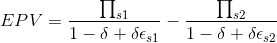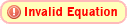# How to decompose the following expression?

• Alec V

#### Alec V

This comes from one of the research papers that I'm reading. The authors decompose Eq. 1 and obtain Eq. 2. After several attempts, I have been fairly unsuccessful at obtaining Eq. 2 from Eq. 1.

I have tried using partial fractions decomposition without much success. Any help would be greatly appreciated.

Eq. 1Eq. 2Thanks!

Try to find the other direction, that is easier (and all steps work both ways). You can simplify equation 2 and get equation 1.

Eq. 2You have to explain the relations that exist among the symbols to get help on this question.

Eq. 2Stephen Tashi said:
You have to explain the relations that exist among the symbols to get help on this question.
Yes, especially ##\Pi_{s1}## and ##\Pi_{sS2}##. Are they just numbers or is something else going on? The symbol ##\Pi## is usually used to denote a product.

Sorry, I should have clarified. ∏s1 is profit in country 1, ∏s2 is profit in country 2, ∈s1 is risk in country 1, ∈s2 is risk in country 2, and δ is the discount rate.

Eq. 2 tries to separate risk elements (∈s1 and ∈s2) from the profit gap between countries. Hope this helps.

∏ as variable is a bit unusual, but as long as all symbols are just variables, their meaning does not matter. This just needs the basic rules to work with fractions.

The results of a simple numerical test did produce identical answers for EPV. (Of course someone should check my program.)

#include <stdio.h>
#include <math.h>

void main()

{

double pi_1, pi_2, delta, ep_1, ep_2;
double frac1,frac2, numerator, denom1, denom2;
double epv_1, epv_2;
pi_1 = 10.0;
pi_2 = 30.0;
delta = 0.25;
ep_1 = 6.0;
ep_2 = 5.0;
printf("pi_1 = %6.3f pi_2 = %6.3f delta = %6.3f ep_1 = %6.3f ep_2 = %6.3f\n\n",pi_1,pi_2,delta,ep_1,ep_2);

denom1 = 1.0 - delta + delta * ep_1;
frac1 = pi_1/denom1;
printf("frac1 %6.4f\n", frac1);
denom2 = 1.0 - delta + delta * ep_2;
frac2 = pi_2/denom2;
printf("frac2 %6.4f\n",frac2);
epv_1 = frac1 - frac2;
printf("epv_1 = %6.3f\n\n",epv_1);

frac1 = (pi_1 - pi_2)/ (1.0 - delta);
printf("frac1 %6.4f\n", frac1);
frac2 = ( delta * ( denom1 * ep_2 * pi_2 - denom2 * ep_1 * pi_1) )/ ((1.0 - delta)*denom1* denom2 );
printf("frac2 %6.4f\n",frac2);
epv_2 = frac1 + frac2;
printf("epv_2 = %6.3f\n", epv_2);

}

The output

pi_1 = 10.000 pi_2 = 30.000 delta = 0.250 ep_1 = 6.000 ep_2 = 5.000

frac1 4.4444
frac2 15.0000
epv_1 = -10.556

frac1 -26.6667
frac2 16.1111
epv_2 = -10.556

Getting rid of terms in a denominator that don't cancel with anything in the numerator is a frequent mathematical daydream. Suppose we approach the problem as the desire to express:

$( \frac{A}{W + F} - \frac{B}{W + G})$ as $( \frac{A}{W} - \frac{B}{W}) \ + \ L$

where $L$ is the leftover stuff.

Then
$L = \frac{A}{W+F} - \frac{A}{W} - \frac{B}{W + G} + \frac{B}{W }$

$= \frac{ AW - A(W+F)} {W(W+F)} + \frac{-BW+B(W+G)}{W(W+G)}$

$= \frac{-AF}{W(W+F)} + \frac{BG}{W(W+G)}$

$= \frac{1}{W} ( \frac{BG}{W+G} - \frac{AF}{W+F})$

In the problem at hand, there are relations between $W, F, G$

$W = 1 -\delta$
$F = \delta \epsilon_1 = (1-W)\epsilon_1$
$G = \delta \epsilon_2 = (1-W)\epsilon_2$

Substituting in selected places for $F$ and $G$

$L = \frac{1}{W}( \frac{B(1-W)\epsilon_2}{W+G} - \frac{A(1-W)\epsilon_1}{W+F} )$

$= \frac{1-W}{W} ( \frac{ \epsilon_2 B}{W+G} - \frac{\epsilon_1 A}{W+F})$

$= \frac{1-W}{W} ( \frac{ (W+F)\epsilon_2 B - (W+G)\epsilon_1A}{(W+G)(W+F)})$

In the problem at hand:
$A = \prod_{s1}$
$B = \prod_{s2}$
$W = (1 -\delta)$
$F = \delta \epsilon_{s1}$
$G = \delta \epsilon_{s2}$
$\epsilon_1 = \epsilon_{s1}$
$\epsilon_2 = \epsilon_{s2}$.

Getting rid of terms in a denominator that don't cancel with anything in the numerator is a frequent mathematical daydream. Suppose we approach the problem as the desire to express:

$( \frac{A}{W + F} - \frac{B}{W + G})$ as $( \frac{A}{W} - \frac{B}{W}) \ + \ L$

where $L$ is the leftover stuff.

Then
$L = \frac{A}{W+F} - \frac{A}{W} - \frac{B}{W + G} + \frac{B}{W }$

$= \frac{ AW - A(W+F)} {W(W+F)} + \frac{-BW+B(W+G)}{W(W+G)}$

$= \frac{-AF}{W(W+F)} + \frac{BG}{W(W+G)}$

$= \frac{1}{W} ( \frac{BG}{W+G} - \frac{AF}{W+F})$

In the problem at hand, there are relations between $W, F, G$

$W = 1 -\delta$
$F = \delta \epsilon_1 = (1-W)\epsilon_1$
$G = \delta \epsilon_2 = (1-W)\epsilon_2$

Substituting in selected places for $F$ and $G$

$L = \frac{1}{W}( \frac{B(1-W)\epsilon_2}{W+G} - \frac{A(1-W)\epsilon_1}{W+F} )$

$= \frac{1-W}{W} ( \frac{ \epsilon_2 B}{W+G} - \frac{\epsilon_1 A}{W+F})$

$= \frac{1-W}{W} ( \frac{ (W+F)\epsilon_2 B - (W+G)\epsilon_1A}{(W+G)(W+F)})$

In the problem at hand:
$A = \prod_{s1}$
$B = \prod_{s2}$
$W = (1 -\delta)$
$F = \delta \epsilon_{s1}$
$G = \delta \epsilon_{s2}$
$\epsilon_1 = \epsilon_{s1}$
$\epsilon_2 = \epsilon_{s2}$.

Thanks so much! The simplification of L makes sense. Could you please dwell on how to separate $( \frac{A}{W} - \frac{B}{W})$ from $( \frac{A}{W + F} - \frac{B}{W + G})$?

. Could you please dwell on how to separate $( \frac{A}{W} - \frac{B}{W})$ from $( \frac{A}{W + F} - \frac{B}{W + G})$?

I'm not sure what you are asking.

I'm not sure what you are asking.

So, we expressed $( \frac{A}{W + F} - \frac{B}{W + G})$ as $( \frac{A}{W} - \frac{B}{W}) \ + \ L$. You then proceed to further simplify the leftover stuff. However, how can we get from $( \frac{A}{W + F} - \frac{B}{W + G})$ to $( \frac{A}{W} - \frac{B}{W}) \ + \ L$?

Thanks.

However, how can we get from $( \frac{A}{W + F} - \frac{B}{W + G})$ to $( \frac{A}{W} - \frac{B}{W}) \ + \ L$?

I just defined $L$ to be $( \frac{A}{W + F} - \frac{B}{W + G}) - ( \frac{A}{W} - \frac{B}{W})$